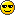# Ch 25.1 Quiz##Ch 25.1 Quiz

Last edited by Kathleen on Thu Apr 16, 2009 10:04 am; edited 1 time in total

##question 3

I have been working on three for a while as well i cant figure it out does anyone know how to solve it? please help!!!!!

sjames
Guest

##question #1

the first equation f=c/lamda, i don't get why we have to do that if we r not using it...and i don't understand how you got to joules because if you multiplied the values to get E, 4.569e-19Js isn't the answer, so i dont really get it at all.

guest 00
Guest

##question 1

i finally figured #1 out..thanks kath..

guest001
Guest

##Question 3

The equation that is on sheet 7 reads
KEmax=e*Vs
we known that e is 1.6e-19 and we are given the stopping voltage(for me) is 6.437 hundred volts.
Since we are asked for the answer in eV and KEmax is normally in joules we can just cancel out the "e" term which is basically like a conversion factor and we just get KEmax=Vs
So to convert to just volts move the decimal place two spots over on the given number
e.g. 643.7 and that is your answer.
The answer is neg.

Guest 24
Guest

##question 1 and 4

I dont understand how to do question 1 or 4 has anyone figured them out?

hwilson
Guest

###1

f=c/lambda (3*10^/GIVEN

super mo
Guest

##question 2 and 4

Did someone one figure out how to do q 2 and 4 because for the life of me I cant!! please help?

john656
Guest

##Maple TA 25_1

A photon has a wavelength of 5.131 x10-7m. What is its energy in eV (sheet 4) ? Indicate with a positive (negative) sign whether the photon energy increases (decreases) with increasing wavelength.

= (3E8)/(5.131E-7)
= 5.84681E14
THEN
=(5.84681E14)*(6.63E-34)
=(3.87644E-19)
THEN
=(3.87644E-19)/(1.6E-19)
=2.42277(NEGATIVE)
The reasoning (step by step):
1. Speed of light divided by the wavelength to get the frequency, and then multiply by "h" which is 6.63E-34 to get the amount of energy in terms of Joules, and then divide by 1.6E-19 in order to convert to eV.

User X
Guest

##Maple TA 25_1

2. A "blackbody" (ideal emissivity - not essential here) emits an electromagnetic spectrum which has its maximum intensity at a wavelength of 587.1 nm. What is the temperature of the backbody in 0C (sheet 4') ? Indicate with a positive (negative) sign whether when heating up a piece of metal and observing the colors of the emitted light the blue (red) light appears first.

= (-2.9E-3)/(587.1E-9)
= -4939.53KELVIN
THEN
=(-4939.53)+(273)
= -4666.53CELSIUS

Reasoning:
The division step gets the temperature in terms of degrees kelvin, but the questions asks for the answer in celsius, so you need to add 273 to that number to get degrees celsius.

User X
Guest

##Maple TA 25_1

I'm having some problems in understanding the concept behind question # 3. If someone could help me understand the rationale behind # 3 please.

User X
Guest

##question 4

I have been working on question four but i cant quite figure it out has anyone figured out how to solve this problem yet??????????

cute611
Guest

##Maple TA 25_1

4. Ok so number 4 is pretty straight forward, it's a simple step that you might have forgotten. So here's the deal:

4. The "workfunction" of a metal emitting electrons via the photoelectric effect is 1.817 eV. What is the threshold frequency i.e. the frequency below which no photoelectrons are emitted (sheet 9) ? Indicate with a positive (negative) sign whether for a certain metal an incident photon in the ultraviolet (infrared) is more likely to release an energetic electron.

= (6.63E-34)/(1.6E-19)
= (4.14375E-15)
THEN
=(1.817)/(4.14375E-15)
=(4.38492E14)

Reasoning: The reason you do the first step is to convert Planck's Constant from Joules into eV(electron-volts).

If someone can please explain the rationale behind question number 3 though, I would appreciate it.

User X
Guest

##Maple TA 25_1

Anyone know how midterm 3 will be spilt up

User X
Guest

##2.

For #2 you have to subtract 273.15 from Kelvin to get Celcius!

Helping
Guest

##Re: Ch 25.1 Quiz

User X wrote:Anyone know how midterm 3 will be spilt up

Midterm 3? You must mean final.

It'll probably be like last semester: 2/3 new material and the remainder review from MT's 1/2.

##Re: Ch 25.1 Quiz